# How do I find the coefficients of static friction and kinetic friction?

• katerowe
The nature of the graph changes quite a bit between the static and kinetic cases. When the object is moving, you'll notice the graph shows the force measurements jumping around a lot. You can describe the data by estimating a value around which the force measurements seems to fluctuate (this would be your best guess for what the actual value of the force kinetic friction is) and a value for how big the fluctuations are (this would be the uncertainty). Can you give us your estimates for those two numbers?The estimates for the two numbers would be something like ##5.5 - 5.7 \pm 0.2 \ N## and ##5.4-5.8 \pm 0.2 \ N##.

#### katerowe

Homework Statement
From a force time graph. Normal force is (18.20 ± 0.01) . I need to find μstatic and μkinetic. I also need to find uncertainties
Relevant Equations
μstatic = F,ax static / F normal
μkinetic = F friction/ F normal
I have found that μstatic= 5.6 (found on graph) / 18.20 = 0.3077
I don't know how to find Force of friction from the graph to calculate μKinetic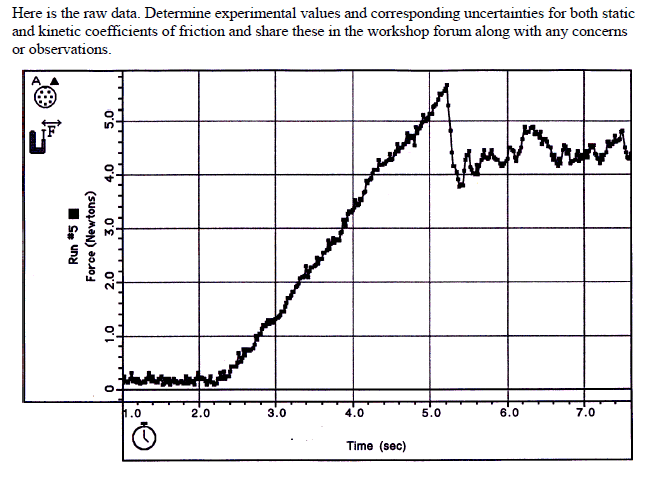#### Attachments

• Friction learning engagement 2-3.pdf
259.1 KB · Views: 77
Last edited by a moderator:
Do the following figures help you understand what you are looking for? Each one is a more ideal representation of the previous one.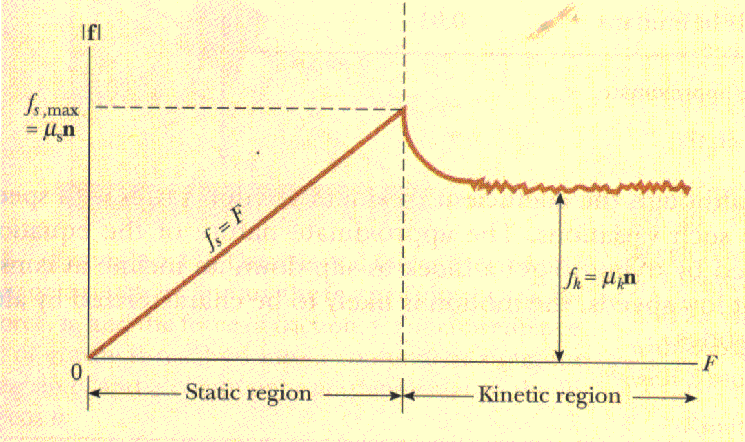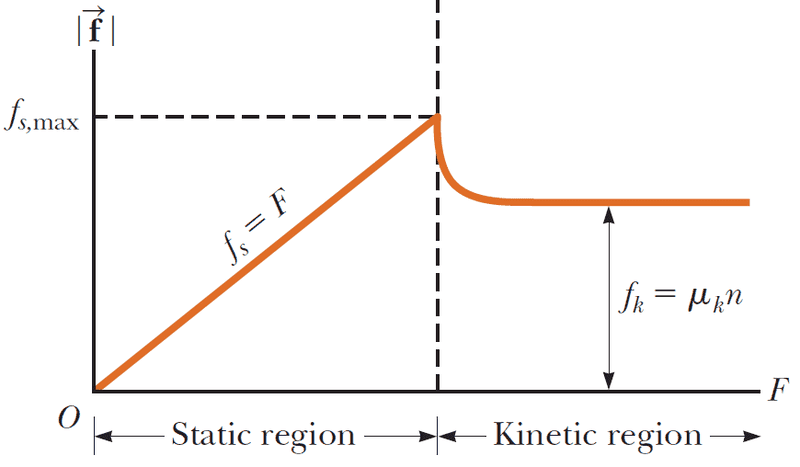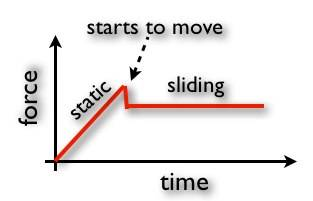•Steve4Physics, jim mcnamara, PeroK and 3 others
Yes, I found both coefficients with μstatic= 0.3077 and μkinetic= 0.2473
Im still unsure how to find the uncertainties. I think I need to divide 0.2/0.1 and thats it but Im not sure

How certain are you that μkinetic = 0.2473 and not something else?

Not very certain

katerowe said:
Not very certain
The graph shows a force of approximately ##5.6 \ N## when the brick starts to move. What is the uncertainty in that value? Could it be ##5.5 - 5.7 \ N##? Or, perhaps, ##5.4-5.8 \ N##?

katerowe said:
Not very certain
The number you gave expresses the value representing the horizontal line in the last figure of my first post. But your data is not a horizontal line. So what is the precision of your value? (Just like the normal force is 18.20 ± 0.01)

0.2 N is the uncertainty?

PeroK said:
The graph shows a force of approximately ##5.6 \ N## when the brick starts to move. What is the uncertainty in that value? Could it be ##5.5 - 5.7 \ N##? Or, perhaps, ##5.4-5.8 \ N##?
0.2N

katerowe said:
0.2N
You mean ##5.6 \pm 0.2 \ N##?

katerowe said:
Yes, I found both coefficients with μstatic= 0.3077 and μkinetic= 0.2473
Im still unsure how to find the uncertainties. I think I need to divide 0.2/0.1 and thats it but Im not sure
It would help if you explained your reasoning here. The expression 0.2/0.1 is meaningless to me.

Your uncertainties will depend on how you determined the coefficients. Can you briefly explain what you did to get your numbers so we don't have to assume?

PeroK said:
You mean ##5.6 \pm 0.2 \ N##?
yes

vela said:
It would help if you explained your reasoning here. The expression 0.2/0.1 is meaningless to me.

Your uncertainties will depend on how you determined the coefficients. Can you briefly explain what you did to get your numbers so we don't have to assume?
0.2 because the scale of the graph goes up by that and 0.1 because that is the uncertainty of normal force

katerowe said:
0.2 because the scale of the graph goes up by that and 0.1 because that is the uncertainty of normal force
That's an uncertainty of ##\pm 2##? Meaning of coefficient of static friction is ##0.3 \pm 2##?

katerowe said:
0.2 because the scale of the graph goes up by that and 0.1 because that is the uncertainty of normal force
You misunderstand how to combine uncertainties. You should have standard formulas for this.
If you know the fractional uncertainties in x and y (δx/x, δy/y) what are the fractional uncertainties in xy and x/y?

katerowe said:
0.2 because the scale of the graph goes up by that
The intervals of the printed gradations is irrelevant. You could draw the same graph but with the gradations at intervals of 0.1N, but it would not change the data, or your calculation of the coefficient or of your confidence in it.
Forget the uncertainty for the moment. How are you estimating the central value for the kinetic coefficient?

katerowe said:
0.2 because the scale of the graph goes up by that and 0.1 because that is the uncertainty of normal force
You're thinking of measuring something with a ruler. In that case, you might estimate the uncertainty based on the size of the divisions. For determining the static coefficient, I wouldn't consider it an unreasonable way to estimate the uncertainty in the ##F_{\rm s,max}## if all you have is the plot. (It sounds like @haruspex might have a better method in mind.)

But the nature of the graph changes quite a bit between the static and kinetic cases. When the object is moving, you'll notice the graph shows the force measurements jumping around a lot. You can describe the data by estimating a value around which the force measurements seems to fluctuate (this would be your best guess for what the actual value of the force kinetic friction is) and a value for how big the fluctuations are (this would be the uncertainty). Can you give us your estimates for those two numbers? You're probably just meant to estimate these values by eye from the plot.

•jack action
vela said:
you might estimate the uncertainty based on the size of the divisions
The divisions on the ruler, yes, but not the divisions printed on the y axis. And that assumes it is clear exactly which point on the plot to use. I want to know what point or points @katerowe intends to use for the kinetic friction.
One thing that bothers me about the question is that we do not know how the force is being measured in the kinetic phase. This could affect how best to estimate the coefficient.

haruspex said:
One thing that bothers me about the question is that we do not know how the force is being measured in the kinetic phase. This could affect how best to estimate the coefficient.
Here is the beginning of the lab writeup taken from the pdf posted by OP. I would assume that the brick is pulled by a force increasing constantly in time until the brick starts moving. The plot is the digitized output of the force sensor. I would trust the data logging interface to record and report accurate time and force measurements.

If I were analyzing this, I would first draw an eyeballed baseline through the fluctuations and call that the force of kinetic friction. It is safe to say that the fluctuations are real and not noise, most probably a manifestation of the stick-slip phenomenon. For the uncertainty in ##\mu_k##, I would draw two parallel lines going through the maximum and minimum values, measure the separation between them and call a fraction of that, say ##\pm \frac{1}{3}\delta##, the uncertainty in the force. Why ##\frac{1}{3}## and not some other fraction? Because it sounds reasonable. Surely, ##\frac{1}{2}## is too much, ##\frac{1}{5}## is not enough and I won't quibble about ##\frac{1}{4}##. This is an estimate after all.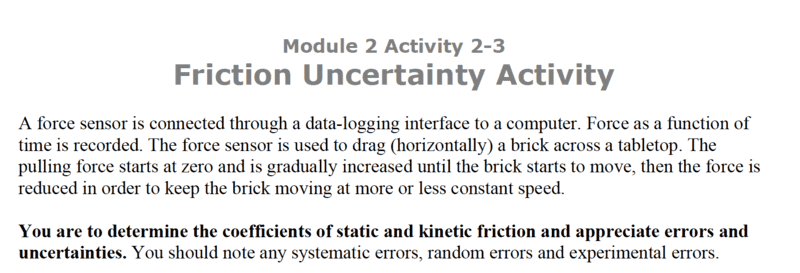kuruman said:
I would trust the data logging interface to record and report accurate time and force measurements.
The extract you posted shows there is a feedback involved. The characteristics of the feedback could be significant.
kuruman said:
the fluctuations are real and not noise, most probably a manifestation of the stick-slip phenomenon
If it were stick-slip I would expect the peak to keep returning to the max static friction force. And we'd better hope it is not or taking the average force in that phase will overestimate the kinetic friction.
If we assume the block never ceases to move and the average acceleration over the kinetic phase is negligible then averaging the force seems ok. But maybe ignore the bit before 6 sec on the basis that the feedback is still adjusting to the rush created by the transition to kinetic.
kuruman said:
Why 1/3 and not some other fraction?
If the data points are available in digital form then how about taking a standard deviation or two?

Ignoring the bit before 6 sec would be a good idea. Taking a standard deviation (or two) would be the way to go but, pending OP's confirmation, the writeup with the plot looks like all that is given.